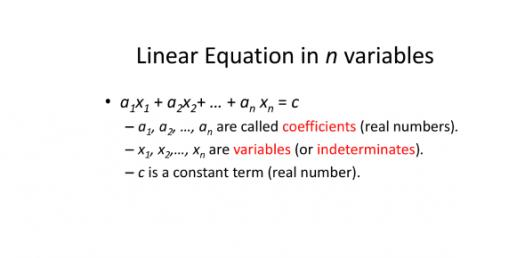Math Quiz: Can You Pass The Linear Equation Test?

9 Questions | Total Attempts: 198SettingsCould you pass the linear equation test? Did you know that an equation is a statement in which the values of two different mathematical equations are identical? Are you able to identify and explain complicated math equations, or do you look at the problem with a giant question mark above your head? Take this quiz and turn a question mark into an exclamation point!

• 1.
Which is the slope - intercept form of a line?
• A.

M = yx + b

• B.

M =(y2-y1) / (x2-x1)

• C.

Y = mx + b

• D.

Y = bx + m

• 2.
What is the slope of the following line? 3x + 7y = 4
• 3.
Determine the slope from the following two points(3,6) and (5,-2)
• 4.
Determine the slope from the following two points.(0,2) and (-4,-2)
• 5.
Rewrite in slope-intercept form.4x - 2y = 6
• 6.
Write the equation in slope-intercept form of the line passing through (-2,4) and has a slope of 3.
• 7.
What is the x-intercept of the equation -2x + 9y = 12
• A.

12/9

• B.

6

• C.

-6

• D.

None of the above

• 8.
Write the equation of the line that is parallel to y = 4x -7 and passes through (1,-3)
• 9.
Evaluate for x = (2,-3,1/2) f(x) = -2x + 3/5
Related TopicsBack to top